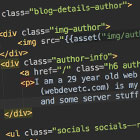## PHP: What is the difference between empty(), isset(), != null, is_null() in PHP

All four of these are ways to basically check if a variable (or return value) is empty or null. But they all have slight differences in how they approach it.

A lot of people assume that `empty()` and `isset()` are opposites, but if you look at the table at the bottom of this page you will see they aren't quite opposite. (e.g. an empty array ([]) will return true for both empty([]) and isset([]) They both also return true for values such as "0", 0, 0.0.

### empty(\$var)

This will check if the variable is empty. The official docs list it as "A variable is considered empty if it does not exist or if its value equals FALSE.".

This means that `empty()` will return true if:

1. if \$var does not exist
2. if \$var = false
3. if \$var = null
4. if \$var = [] (empty array)
5. if \$var = "" (empty string)
6. if \$var = 0
7. if \$var = "0" (0 as a string)
8. if \$var = 0.0 (0 as a float)

### isset(\$val)

`isset()` is a mix between checking if something is set (i.e. the variable exists, or in the case of arrays if the array key exists)) and checking if it is not null

It will return true for most situations, unless the variable does not exist, or if the variable = null

If you supply multiple arguments, it will only return true if all are set.

`\$a  = \$b = \$c = true;\$null1 = \$null2 = null; var_dump( [    "a_b_c" => isset(\$a,\$b,\$c),    "null1_null2" => isset(\$null1,\$null2),    "a_b_null1" => isset(\$a,\$b,\$null1),]);`
//\$a = \$b = \$c = true; //\$null1 = \$null2 = null; // // echo "
```";
//var_dump( [
//
//        "a_b_c" => isset(\$a,\$b,\$c),
//        "null1_null2" => isset(\$null1,\$null2),
//        "a_b_null1" => isset(\$a,\$b,\$null1),
//
//]);
//        echo "```
";// \$a = ) ?>
```array(3) {
["a b c"]=> bool(true) // all were set and have values
["null1 null2"]=> bool(false) // both were null
["a b null1"]=> bool(false) // one was null
}```

### \$val == null

This will return true for all situations where \$val has a value. There are some weird situations that you must be aware of though.

It will return false for `\$val = "0"; var_dump(\$val == null); `. I don't know why.

### \$val === null and is_null(\$val)

The only time that either of these statements will return true is if \$val actually is null (i.e. \$val=null;).

Testempty(\$val)isset(\$val)\$val == null\$val === nullis_null(\$val)
false true true true false false
true false true false false false
null true false true true true
empty array true true true false false
array with 1 item false true false false false
empty string ("") true true true false false
space (" ") false true false false false
0 true true true false false
"0" (as string) true true false false false
0.0 (as float) true true true false falsewebdevetc

I am a 29 year old backend web developer from London, mostly focusing on PHP and Laravel lately. This (webdevetc.com) is my blog where I write about some web development topics (PHP, Laravel, Javascript, and some server stuff). contact me here.

### More...

##### Or see other languages/frameworks:
PHP Laravel Composer Apache CentOS and Linux Stuff WordPress General Webdev and Programming Stuff JavaScript